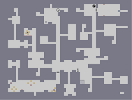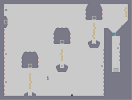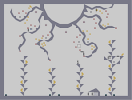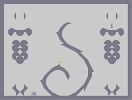### Death-Kid-kunHover over the thumbnail for a full-size version.

Author darkcrusader author:darkcrusader manga mines n-art non-playable rated soul-eater 2010-04-02 2010-04-02 4 by 27 people. \$Death-Kid-kun#darkcrusader#non-playable#00000000000000000000000000000000000000000000000000000000000000000000000000000000000000000000000000000000000000000000000000000000000000000000000000000000000000000000000000000000000000000000000000000000000000000000000000000000000000000000000000000000000000000000000000000000000000000000000000000000000000000000000000000000000000000000000000000000000000000000000000000000000000000000000000000000000000000000000000000000000000000000000000000000000000000000000000000000000000000000000000000000000000000000000000000000000000000000000000000000000000000000000000000000000000000000000000000000000000000000000000000000000000000000000000000000000000000000000000000000000000000000000000000000000000000000000000000000000000000|12^694,201!12^664,88!12^694,139!12^608,49!12^529,31!12^405,85!12^449,51!12^362,151!12^382,112!12^425,64!12^639,68!12^683,111!12^696,167!12^342,182!12^325,208!12^362,195!12^345,201!12^377,185!12^394,195!12^370,202!12^359,222!12^349,249!12^352,238!12^381,208!12^373,217!12^363,230!12^354,240!12^365,212!12^356,229!12^374,192!12^367,259!12^377,235!12^387,216!12^391,202!12^382,225!12^370,247!12^453,160!12^397,225!12^383,242!12^388,235!12^376,251!12^406,213!12^433,183!12^443,170!12^425,190!12^450,239!12^498,160!12^470,196!12^452,193!12^453,177!12^460,216!12^503,343!12^491,265!12^483,176!12^496,174!12^490,226!12^495,304!12^578,180!12^583,268!12^583,214!12^584,240!12^581,193!12^600,216!12^589,240!12^582,263!12^582,256!12^583,247!12^587,245!12^586,252!12^585,257!12^584,262!12^582,251!12^583,239!12^583,232!12^583,230!12^583,222!12^583,218!12^591,237!12^593,231!12^596,225!12^598,219!12^605,326!12^606,254!12^606,282!12^605,230!12^602,211!12^603,219!12^604,224!12^606,249!12^606,243!12^606,235!12^607,240!12^606,276!12^605,270!12^606,260!12^606,267!12^606,264!12^605,322!12^605,317!12^605,312!12^605,309!12^605,304!12^605,299!12^605,290!12^605,296!12^606,285!12^649,217!12^665,278!12^663,284!12^629,293!12^629,301!12^630,289!12^632,284!12^634,277!12^638,264!12^640,258!12^642,252!12^645,245!12^646,238!12^647,235!12^648,230!12^649,224!12^635,274!12^637,271!12^616,311!12^614,315!12^611,319!12^609,323!12^620,306!12^622,302!12^624,297!12^627,293!12^581,199!12^582,208!12^582,202!12^579,189!12^579,180!12^579,185!12^573,182!12^570,185!12^566,188!12^562,192!12^557,197!12^540,236!12^539,241!12^543,228!12^545,221!12^548,215!12^550,209!12^554,202!12^538,247!12^536,254!12^534,262!12^532,268!12^531,275!12^529,283!12^530,281!12^528,287!12^526,294!12^524,300!12^523,307!12^521,313!12^518,321!12^516,328!12^516,332!12^515,340!12^514,345!12^514,355!12^514,351!12^513,365!12^513,359!12^510,359!12^504,349!12^507,355!12^498,322!12^493,281!12^490,243!12^492,208!12^493,190!12^501,338!12^500,332!12^499,328!12^497,316!12^495,309!12^493,291!12^493,285!12^494,297!12^491,271!12^492,276!12^490,260!12^490,255!12^489,252!12^489,249!12^490,239!12^490,234!12^490,230!12^491,220!12^491,216!12^491,212!12^493,203!12^493,199!12^493,196!12^495,183!12^495,178!12^498,169!12^498,164!12^496,163!12^491,166!12^487,169!12^485,172!12^481,180!12^478,183!12^476,186!12^472,192!12^474,189!12^468,199!12^466,203!12^464,206!12^463,210!12^455,227!12^458,220!12^456,224!12^454,230!12^453,235!12^452,207!12^452,216!12^451,224!12^451,234!12^450,229!12^451,221!12^451,211!12^451,202!12^452,196!12^452,188!12^452,182!12^453,175!12^453,168!12^453,165!12^684,302!12^683,308!12^632,340!12^631,333!12^631,327!12^630,321!12^630,316!12^629,313!12^628,308!12^628,305!12^637,340!12^634,347!12^641,336!12^645,329!12^647,322!12^650,317!12^654,310!12^657,303!12^659,298!12^662,289!12^660,295!12^682,314!12^623,367!12^629,369!12^634,371!12^648,363!12^666,339!12^675,326!12^658,350!12^680,320!12^678,323!12^672,331!12^669,336!12^664,343!12^660,347!12^655,354!12^652,359!12^646,366!12^643,369!12^637,371!12^666,296!12^673,298!12^673,305!12^672,311!12^670,317!12^634,353!12^641,353!12^645,349!12^649,345!12^653,341!12^656,337!12^661,331!12^666,324!12^666,344!12^707,340!12^699,345!12^693,347!12^686,348!12^678,349!12^671,347!12^682,349!12^665,384!12^691,370!12^705,355!12^712,336!12^705,343!12^712,341!12^710,344!12^708,350!12^702,360!12^698,365!12^694,368!12^688,372!12^685,375!12^682,376!12^677,379!12^672,382!12^696,391!12^692,393!12^684,393!12^679,393!12^673,391!12^667,388!12^689,394!12^632,407!12^693,394!12^689,397!12^666,407!12^657,408!12^651,408!12^644,408!12^636,407!12^661,408!12^670,406!12^687,400!12^682,403!12^677,405!12^626,408!12^620,405!12^611,403!12^614,405!12^606,402!12^620,370!12^617,375!12^614,379!12^611,385!12^608,390!12^605,393!12^602,397!12^599,402!12^593,406!12^587,410!12^581,413!12^448,163!12^443,167!12^439,174!12^436,178!12^428,187!12^423,193!12^419,199!12^415,204!12^411,208!12^409,210!12^404,215!12^399,222!12^401,219!12^394,229!12^419,197!12^392,232!12^385,239!12^379,246!12^372,255!12^368,258!12^364,262!12^367,254!12^369,250!12^373,244!12^375,239!12^379,231!12^385,220!12^387,213!12^389,206!12^394,199!12^390,200!12^387,203!12^383,205!12^379,209!12^376,212!12^374,214!12^370,220!12^367,223!12^364,226!12^359,233!12^355,236!12^351,246!12^351,242!12^358,227!12^362,217!12^366,207!12^371,198!12^376,189!12^374,187!12^370,190!12^366,193!12^358,197!12^352,199!12^348,201!12^340,203!12^336,205!12^331,207!12^677,271!12^679,267!12^680,263!12^682,257!12^683,249!12^326,204!12^330,200!12^334,195!12^336,191!12^340,185!12^337,189!12^363,252!12^361,249!12^358,245!12^364,265!12^360,269!12^349,254!12^350,260!12^352,265!12^354,270!12^354,274!12^351,286!12^352,282!12^348,302!12^350,292!12^349,298!12^347,310!12^348,307!12^353,278!12^346,316!12^345,322!12^346,332!12^346,327!12^350,348!12^347,338!12^348,344!12^352,352!12^354,357!12^356,362!12^373,391!12^369,385!12^365,380!12^363,376!12^360,371!12^358,368!12^396,412!12^409,421!12^384,400!12^407,421!12^402,417!12^399,414!12^395,412!12^390,408!12^388,405!12^381,399!12^377,395!12^413,425!12^416,429!12^450,430!12^445,430!12^441,430!12^432,430!12^425,430!12^420,430!12^436,431!12^453,432!12^576,415!12^567,418!12^561,421!12^556,423!12^549,425!12^545,428!12^541,430!12^535,432!12^529,434!12^525,436!12^520,437!12^518,438!12^514,440!12^510,441!12^506,443!12^427,409!12^483,411!12^434,408!12^440,407!12^447,406!12^467,407!12^479,411!12^475,409!12^470,408!12^460,405!12^456,405!12^452,405!12^478,342!12^475,339!12^472,336!12^469,333!12^467,331!12^462,328!12^458,327!12^454,328!12^453,331!12^396,232!12^442,259!12^401,235!12^405,238!12^408,240!12^438,256!12^432,253!12^427,250!12^422,246!12^417,244!12^413,242!12^446,262!12^447,264!12^398,241!12^396,246!12^448,275!12^450,273!12^453,271!12^453,269!12^449,266!12^445,264!12^408,246!12^407,253!12^407,259!12^411,264!12^416,266!12^416,268!12^434,269!12^429,270!12^424,270!12^419,250!12^424,259!12^426,256!12^423,255!12^419,254!12^422,256!12^425,282!12^418,281!12^411,278!12^406,274!12^399,270!12^395,253!12^395,258!12^395,260!12^397,266!12^403,274!12^452,263!12^448,261!12^444,259!12^440,255!12^436,252!12^468,290!12^467,286!12^464,276!12^462,270!12^457,267!12^459,264!12^457,260!12^421,200!12^457,257!12^449,243!12^445,237!12^442,232!12^439,228!12^436,225!12^432,220!12^428,215!12^424,212!12^419,207!12^456,254!12^453,249!12^455,256!12^451,251!12^450,249!12^447,244!12^444,240!12^441,236!12^439,231!12^437,230!12^431,223!12^466,284!12^465,277!12^474,289!12^471,286!12^469,282!12^467,278!12^465,272!12^462,268!12^462,262!12^465,270!12^467,273!12^468,275!12^470,278!12^472,282!12^474,287!12^463,267!12^459,259!12^483,273!12^484,278!12^484,284!12^482,287!12^479,292!12^476,294!12^400,231!12^403,233!12^405,234!12^408,236!12^449,261!12^444,258!12^442,255!12^437,251!12^431,248!12^425,245!12^422,243!12^418,240!12^413,238!12^438,270!12^402,270!12^408,264!12^396,264!12^441,265!12^429,256!12^414,258!12^432,264!12^501,446!12^460,435!12^494,445!12^488,443!12^484,442!12^479,440!12^474,439!12^468,438!12^464,435!12^457,434!12^538,459!12^531,457!12^526,456!12^524,455!12^520,453!12^515,451!12^508,450!12^558,465!12^553,464!12^547,462!12^542,461!12^563,467!12^585,501!12^574,477!12^567,469!12^570,472!12^581,489!12^586,517!12^584,530!12^585,527!12^586,522!12^586,513!12^585,510!12^585,506!12^584,496!12^580,487!12^578,483!12^583,534!12^531,563!12^507,556!12^438,538!12^473,547!12^527,562!12^520,560!12^513,558!12^503,555!12^496,553!12^489,550!12^481,548!12^467,545!12^459,544!12^454,541!12^447,540!12^430,536!12^423,534!12^415,532!12^561,559!12^546,564!12^574,548!12^581,540!12^578,544!12^570,553!12^566,557!12^558,561!12^553,563!12^542,564!12^535,563!12^544,477!12^561,517!12^559,493!12^543,535!12^520,537!12^504,519!12^507,494!12^521,481!12^531,476!12^554,484!12^562,503!12^554,528!12^532,539!12^509,530!12^503,506!12^512,487!12^516,484!12^526,478!12^537,475!12^549,480!12^557,489!12^561,499!12^563,508!12^562,513!12^559,520!12^557,524!12^551,531!12^548,533!12^540,537!12^537,539!12^529,540!12^523,538!12^518,537!12^512,533!12^506,525!12^502,513!12^504,500!12^509,490!12^493,500!12^500,483!12^495,491!12^498,486!12^494,496!12^508,475!12^516,470!12^524,467!12^537,467!12^530,466!12^511,472!12^504,478!12^559,477!12^554,474!12^548,470!12^544,468!12^564,483!12^569,489!12^571,494!12^573,501!12^573,506!12^573,512!12^572,517!12^571,524!12^569,529!12^567,533!12^565,537!12^562,540!12^559,543!12^554,546!12^549,548!12^520,468!12^544,550!12^539,551!12^537,551!12^531,551!12^524,550!12^518,548!12^512,546!12^507,544!12^503,540!12^499,537!12^496,533!12^494,529!12^492,527!12^492,524!12^492,520!12^491,518!12^491,512!12^491,508!12^491,504!12^391,517!12^397,520!12^401,523!12^406,526!12^410,529!12^421,533!12^443,539!12^411,429!12^408,433!12^405,435!12^390,513!12^390,508!12^389,505!12^384,503!12^378,501!12^373,500!12^364,496!12^369,498!12^400,434!12^393,432!12^385,430!12^379,428!12^371,425!12^337,448!12^341,437!12^348,428!12^357,424!12^365,423!12^339,441!12^344,432!12^353,426!12^361,424!12^361,495!12^356,492!12^350,490!12^345,487!12^340,484!12^337,479!12^336,476!12^334,460!12^335,454!12^377,442!12^369,481!12^352,458!12^355,469!12^381,479!12^393,467!12^388,450!12^363,445!12^362,477!12^374,481!12^384,477!12^389,472!12^392,461!12^390,455!12^385,447!12^382,445!12^359,448!12^354,453!12^353,464!12^359,473!12^369,443!12^372,442!12^590,529!12^598,531!12^605,534!12^611,537!12^613,540!12^612,545!12^611,548!12^611,553!12^609,555!12^606,556!12^603,554!12^600,554!12^597,553!12^592,551!12^589,551!12^585,551!12^582,549!12^604,559!12^600,564!12^596,566!12^594,569!12^593,572!12^578,553!12^574,560!12^571,565!12^568,572!12^566,575!12^386,509!12^384,514!12^383,520!12^381,525!12^378,532!12^378,538!12^376,544!12^375,552!12^373,559!12^373,565!12^371,567!12^370,571!12^399,526!12^396,532!12^393,536!12^390,540!12^387,545!12^384,548!12^381,553!12^377,559!12^376,548!12^335,471!12^332,473!12^332,478!12^332,483!12^332,487!12^332,494!12^377,522!12^370,520!12^366,519!12^361,517!12^356,514!12^349,512!12^344,509!12^341,506!12^337,504!12^334,501!12^327,480!12^324,483!12^319,488!12^319,493!12^320,501!12^374,534!12^368,532!12^362,530!12^356,528!12^352,526!12^348,525!12^343,523!12^338,521!12^333,518!12^326,512!12^322,507!12^330,517!12^319,493!12^318,497!12^318,503!12^316,513!12^314,520!12^314,525!12^314,531!12^312,534!12^312,538!12^312,543!12^310,549!12^310,553!12^308,558!12^308,562!12^308,563!12^308,563!12^308,568!12^308,569!12^316,509!12^318,486!12^248,459!12^281,472!12^299,479!12^263,465!12^314,485!12^309,483!12^304,481!12^294,478!12^287,475!12^275,469!12^270,468!12^258,462!12^252,461!12^236,479!12^313,506!12^274,492!12^295,500!12^254,485!12^307,504!12^300,503!12^290,499!12^283,496!12^278,494!12^268,490!12^261,487!12^247,483!12^241,481!12^245,462!12^243,466!12^239,471!12^238,474!12^712,506!12^668,450!12^623,411!12^647,428!12^693,477!12^704,492!12^680,462!12^657,437!12^636,419!12^720,533!12^717,554!12^711,573!12^714,566!12^716,560!12^719,549!12^720,544!12^720,538!12^720,530!12^719,527!12^717,523!12^717,519!12^716,516!12^714,511!12^710,501!12^708,497!12^701,487!12^698,482!12^690,472!12^684,466!12^687,469!12^678,460!12^675,457!12^672,454!12^667,449!12^665,446!12^661,440!12^655,435!12^652,431!12^645,427!12^642,424!12^639,422!12^631,415!12^354,273!12^351,265!12^349,271!12^343,269!12^336,264!12^330,262!12^326,259!12^323,257!12^320,254!12^340,267!12^317,251!12^314,248!12^311,245!12^321,287!12^318,283!12^315,279!12^313,274!12^312,271!12^310,267!12^310,264!12^309,256!12^309,254!12^308,251!12^307,247!12^306,244!12^306,240!12^287,275!12^292,277!12^298,280!12^304,282!12^310,283!12^317,286!12^316,285!12^343,334!12^340,332!12^337,329!12^300,298!12^294,294!12^292,292!12^287,284!12^281,279!12^275,274!12^283,274!12^303,302!12^288,288!12^283,282!12^326,321!12^320,317!12^315,314!12^311,310!12^307,306!12^333,326!12^329,323!12^279,275!12^684,252!12^685,247!12^686,241!12^687,240!12^688,233!12^689,228!12^691,220!12^692,213!12^693,209!12^693,205!12^695,195!12^695,191!12^695,185!12^696,185!12^696,181!12^696,178!12^696,176!12^696,173!12^696,161!12^696,157!12^695,151!12^695,147!12^695,145!12^692,134!12^691,130!12^689,126!12^689,122!12^687,120!12^687,116!12^681,105!12^678,101!12^675,98!12^672,95!12^668,91!12^660,84!12^657,81!12^655,79!12^652,77!12^645,72!12^643,70!12^635,65!12^631,62!12^628,58!12^624,57!12^619,53!12^615,51!12^649,74!12^604,47!12^598,43!12^592,40!12^586,38!12^579,36!12^571,33!12^577,34!12^541,29!12^535,29!12^565,31!12^552,29!12^546,29!12^559,29!12^523,30!12^516,31!12^510,31!12^505,32!12^499,33!12^492,34!12^487,36!12^481,37!12^473,41!12^462,45!12^453,48!12^457,47!12^468,42!12^476,39!12^445,52!12^439,56!12^430,62!12^433,60!12^422,67!12^418,71!12^415,74!12^411,78!12^407,83!12^403,86!12^399,90!12^395,94!12^392,99!12^387,104!12^384,107!12^388,102!12^381,113!12^379,119!12^375,124!12^373,129!12^369,134!12^367,138!12^364,144!12^359,157!12^355,161!12^344,178!12^346,174!12^348,171!12^351,168!12^352,166!12^583,73!12^621,82!12^686,119!12^684,118!12^668,106!12^654,97!12^677,112!12^599,75!12^692,156!12^661,135!12^678,144!12^639,126!12^616,117!12^589,111!12^572,95!12^576,110!12^602,113!12^627,121!12^650,131!12^669,139!12^686,149!12^662,101!12^633,87!12^611,78!12^644,92!12^569,108!12^583,111!12^596,111!12^610,116!12^622,119!12^631,122!12^645,128!12^656,134!12^689,153!12^684,148!12^674,142!12^668,137!12^577,87!12^581,76!12^595,74!12^589,73!12^607,78!12^602,76!12^616,80!12^627,84!12^640,89!12^649,95!12^658,99!12^673,108!12^667,103!12^575,89!12^579,81!12^691,212!12^544,168!12^696,180!12^637,153!12^594,137!12^673,173!12^562,171!12^577,176!12^550,170!12^557,171!12^568,173!12^574,173!12^570,100!12^569,103!12^558,130!12^556,136!12^554,142!12^552,146!12^550,151!12^549,155!12^546,160!12^543,164!12^563,130!12^570,130!12^583,133!12^589,135!12^577,131!12^634,151!12^628,149!12^625,147!12^619,145!12^613,143!12^606,140!12^600,137!12^669,171!12^663,167!12^659,165!12^652,162!12^646,158!12^643,155!12^691,186!12^684,181!12^678,176!12^689,183!12^689,224!12^688,215!12^682,213!12^678,211!12^656,200!12^597,178!12^674,209!12^666,206!12^661,203!12^657,201!12^651,197!12^645,195!12^640,192!12^634,189!12^629,188!12^623,185!12^617,182!12^612,181!12^608,179!12^603,178!12^598,177!12^594,176!12^590,175!12^587,175!12^583,173!12^579,173!12^683,296!12^671,283!12^679,291!12^676,285!12^675,276!12^672,276!12^681,252!12^678,254!12^673,254!12^666,254!12^660,255!12^656,255!12^651,255!12^646,253!12^643,253!12^631,253!12^621,253!12^617,253!12^611,253!12^626,254!12^637,254!12^685,228!12^677,230!12^670,230!12^663,228!12^657,228!12^652,227!12^646,224!12^607,215!12^619,217!12^644,224!12^640,224!12^634,222!12^631,222!12^624,220!12^619,220!12^616,219!12^612,219!12^598,212!12^591,209!12^550,201!12^543,200!12^537,197!12^534,197!12^532,201!12^529,207!12^529,209!12^527,213!12^527,217!12^525,220!12^524,225!12^521,231!12^519,235!12^519,240!12^530,244!12^518,244!12^526,244!12^531,246!12^534,248!12^521,244!12^586,209!12^595,209!12^600,308!12^592,306!12^583,303!12^572,299!12^567,298!12^577,301!12^587,305!12^595,307!12^598,312!12^597,319!12^539,312!12^542,316!12^545,320!12^549,324!12^554,326!12^559,330!12^594,324!12^600,306!12^597,305!12^591,303!12^589,302!12^583,300!12^578,300!12^573,298!12^570,297!12^563,293!12^560,292!12^566,295!12^575,320!12^587,311!12^583,315!12^579,318!12^570,320!12^566,319!12^563,319!12^559,317!12^556,314!12^547,304!12^552,311!12^550,307!12^548,302!12^547,298!12^547,293!12^549,291!12^549,288!12^554,288!12^573,304!12^571,307!12^566,308!12^564,306!12^560,303!12^560,298!12^565,304!12^565,302!12^555,290!12^538,308!12^536,304!12^536,299!12^602,272!12^595,273!12^589,274!12^581,275!12^574,278!12^568,279!12^563,280!12^556,281!12^554,282!12^547,283!12^544,286!12^540,288!12^536,291!12^533,295!12^534,290!12^537,286!12^540,283!12^546,280!12^551,280!12^554,280!12^555,278!12^559,278!12^564,276!12^569,276!12^576,312!12^564,312!12^552,300!12^588,327!12^584,329!12^580,331!12^573,332!12^566,331!12^288,295!12^284,295!12^278,295!12^200,301!12^240,293!12^274,294!12^269,294!12^261,293!12^256,293!12^249,292!12^244,292!12^234,292!12^227,293!12^219,294!12^212,296!12^207,297!12^203,300!12^195,303!12^188,304!12^182,307!12^177,310!12^170,313!12^164,317!12^160,321!12^155,325!12^143,343!12^145,338!12^152,328!12^309,526!12^302,522!12^298,519!12^294,516!12^289,513!12^284,511!12^277,507!12^273,507!12^265,504!12^261,503!12^250,498!12^255,500!12^268,507!12^230,500!12^231,493!12^232,491!12^235,485!12^228,550!12^228,545!12^227,545!12^227,541!12^227,539!12^228,536!12^228,532!12^228,528!12^228,523!12^228,517!12^228,513!12^229,508!12^229,504!12^248,502!12^246,505!12^244,511!12^244,516!12^244,521!12^243,525!12^243,528!12^243,532!12^243,535!12^243,539!12^243,542!12^243,546!12^243,549!12^243,556!12^228,555!12^245,559!12^250,562!12^257,565!12^265,569!12^272,572!12^280,573!12^302,569!12^294,571!12^281,573!12^305,572!12^287,572!12^282,567!12^273,568!12^251,525!12^252,530!12^252,535!12^252,542!12^262,565!12^258,563!12^256,559!12^255,555!12^254,553!12^253,549!12^286,525!12^282,524!12^274,523!12^267,523!12^260,525!12^257,527!12^583,417!12^578,429!12^573,433!12^569,435!12^563,436!12^555,438!12^550,440!12^543,442!12^538,445!12^532,445!12^525,445!12^522,445!12^515,445!12^341,339!12^334,346!12^332,349!12^337,343!12^332,352!12^332,359!12^334,363!12^339,371!12^345,377!12^351,384!12^356,390!12^362,399!12^363,406!12^366,403!12^370,400!12^373,394!12^359,393!12^354,386!12^347,380!12^342,374!12^337,368!12^355,400!12^350,396!12^345,394!12^341,394!12^333,392!12^329,392!12^323,391!12^318,391!12^313,392!12^308,394!12^252,519!12^252,512!12^253,508!12^287,561!12^283,565!12^365,412!12^350,423!12^383,425!12^377,419!12^373,416!12^370,414!12^367,411!12^366,416!12^364,421!12^262,460!12^302,529!12^301,535!12^301,540!12^301,544!12^300,551!12^299,557!12^296,561!12^293,563!12^290,564!12^323,479!12^328,472!12^333,465!12^331,467!12^328,476!12^299,411!12^296,413!12^293,417!12^288,421!12^285,424!12^281,428!12^278,430!12^274,433!12^270,439!12^265,459!12^265,454!12^267,451!12^266,446!12^268,442!12^271,437!12^309,391!12^305,390!12^300,388!12^293,386!12^287,385!12^281,384!12^274,382!12^270,379!12^261,377!12^289,527!12^291,528!12^290,536!12^290,547!12^287,555!12^290,542!12^288,551!12^266,379!12^258,378!12^251,378!12^248,383!12^244,389!12^190,439!12^196,435!12^200,430!12^209,422!12^214,418!12^218,414!12^222,411!12^226,408!12^229,406!12^232,402!12^235,398!12^239,395!12^205,425!12^166,522!12^166,514!12^167,507!12^167,500!12^169,494!12^169,488!12^170,482!12^170,478!12^171,470!12^172,464!12^185,443!12^182,445!12^177,449!12^175,454!12^172,458!12^168,529!12^172,537!12^174,542!12^176,547!12^178,552!12^180,559!12^184,565!12^185,568!12^228,561!12^226,565!12^228,571!12^232,574!12^188,574!12^244,383!12^238,387!12^232,390!12^228,390!12^224,387!12^217,387!12^210,384!12^204,383!12^198,383!12^195,386!12^189,390!12^220,410!12^215,407!12^211,404!12^204,400!12^200,398!12^194,396!12^187,396!12^189,394!12^197,395!12^205,398!12^219,408!12^215,405!12^211,402!12^207,401!12^184,397!12^180,394!12^175,397!12^171,402!12^167,408!12^163,412!12^160,415!12^157,418!12^165,417!12^173,413!12^177,411!12^183,418!12^181,423!12^181,427!12^181,432!12^182,433!12^182,434!12^182,437!12^170,416!12^183,411!12^154,421!12^150,425!12^145,428!12^138,433!12^133,436!12^130,439!12^126,448!12^124,455!12^123,462!12^122,469!12^121,476!12^120,483!12^120,492!12^120,500!12^120,508!12^120,513!12^120,521!12^120,525!12^120,529!12^119,536!12^122,541!12^124,548!12^130,555!12^135,560!12^138,566!12^142,571!12^146,576!12^165,392!12^173,392!12^167,393!12^157,393!12^153,393!12^149,393!12^144,393!12^139,393!12^129,393!12^121,394!12^115,394!12^125,395!12^474,576!12^477,572!12^481,572!12^484,573!12^488,573!12^491,574!12^494,577!12^496,576!12^501,576!12^133,394!12^110,397!12^105,402!12^103,409!12^101,416!12^100,422!12^98,428!12^95,437!12^94,443!12^93,448!12^92,452!12^97,432!12^91,458!12^89,465!12^87,472!12^83,480!12^83,487!12^84,497!12^85,504!12^88,511!12^89,520!12^92,526!12^93,532!12^94,534!12^98,545!12^98,548!12^101,554!12^106,554!12^116,554!12^119,550!12^93,528!12^97,525!12^102,522!12^109,522!12^113,525!12^116,525!12^98,542!12^94,539!12^85,477!12^84,493!12^94,427!12^87,429!12^78,430!12^73,430!12^66,430!12^61,430!12^54,431!12^82,430!12^48,430!12^42,436!12^44,430!12^39,438!12^34,444!12^28,449!12^25,457!12^92,435!12^88,435!12^85,435!12^81,437!12^80,438!12^77,441!12^73,444!12^69,451!12^66,455!12^62,459!12^58,466!12^54,470!12^52,475!12^50,478!12^46,481!12^429,283!12^437,282!12^444,280!12^43,486!12^36,491!12^30,496!12^30,502!12^30,507!12^30,511!12^30,517!12^92,544!12^87,542!12^82,541!12^72,537!12^67,537!12^62,536!12^57,533!12^51,532!12^44,529!12^38,527!12^30,525!12^81,496!12^77,500!12^73,504!12^69,506!12^74,511!12^78,513!12^85,514!12^73,539!12^77,542!12^83,509!12^140,350!12^139,355!12^137,362!12^136,368!12^134,391!12^131,380!12^129,385!12^129,388!12^134,373!12^138,576!12^132,576!12^132,570!12^138,570!12^132,564!12^126,564!12^126,558!12^126,552!12^132,558!12^120,558!12^114,558!12^108,558!12^120,564!12^114,564!12^108,564!12^108,570!12^114,570!12^120,570!12^126,570!12^126,576!12^114,576!12^120,576!12^108,576!12^102,576!12^102,570!12^102,564!12^102,558!12^96,558!12^96,552!12^96,564!12^96,570!12^96,576!12^90,576!12^90,570!12^90,564!12^90,558!12^90,552!12^90,546!12^84,546!12^84,552!12^84,558!12^84,564!12^84,570!12^84,576!12^78,576!12^78,570!12^78,564!12^78,558!12^78,552!12^78,546!12^72,546!12^72,552!12^72,558!12^72,564!12^72,570!12^72,576!12^66,576!12^66,570!12^66,564!12^66,558!12^66,552!12^66,546!12^66,540!12^60,540!12^60,546!12^60,552!12^60,558!12^60,564!12^60,570!12^60,570!12^60,576!12^54,576!12^54,570!12^54,564!12^54,558!12^54,552!12^54,546!12^54,540!12^48,534!12^48,540!12^48,546!12^48,552!12^48,558!12^48,564!12^48,570!12^48,576!12^42,576!12^42,570!12^42,564!12^42,558!12^42,552!12^42,546!12^42,540!12^42,534!12^36,534!12^36,540!12^36,546!12^36,552!12^36,558!12^36,564!12^36,570!12^36,576!12^30,576!12^30,564!12^30,570!12^24,570!12^24,576!12^24,564!12^30,558!12^24,558!12^30,552!12^24,552!12^24,546!12^30,546!12^30,540!12^24,540!12^30,528!12^24,534!12^30,534!12^24,528!12^24,522!12^24,516!12^24,510!12^24,504!12^24,498!12^84,534!12^90,528!12^78,528!12^72,534!12^84,522!12^72,522!12^78,516!12^66,528!12^60,534!12^54,528!12^60,522!12^48,522!12^36,522!12^36,510!12^42,516!12^48,510!12^54,516!12^60,510!12^66,516!12^54,504!12^42,504!12^36,498!12^48,498!12^24,492!12^30,492!12^66,438!12^66,432!12^66,444!12^72,444!12^60,444!12^54,444!12^54,438!12^54,432!12^60,432!12^48,444!12^42,444!12^42,438!12^36,444!12^42,450!12^42,456!12^48,456!12^54,456!12^54,450!12^60,456!12^66,450!12^42,462!12^42,468!12^48,468!12^54,468!12^54,462!12^42,474!12^42,480!12^36,480!12^30,480!12^30,474!12^30,468!12^36,468!12^30,462!12^30,456!12^36,456!12^30,450!12^24,468!12^24,480!12^30,486!12^39,490!12^552,570!12^540,570!12^546,576!12^534,576!12^528,570!12^522,576!12^516,570!12^522,564!12^510,564!12^510,576!12^504,570!12^492,570!12^498,564!12^504,558!12^492,558!12^486,564!12^480,558!12^474,564!12^486,552!12^468,558!12^474,546!12^474,552!12^468,570!12^462,576!12^456,570!12^462,564!12^456,558!12^462,552!12^456,546!12^468,546!12^450,564!12^450,576!12^444,570!12^450,552!12^444,558!12^444,546!12^438,552!12^438,564!12^438,576!12^432,570!12^432,558!12^432,546!12^438,540!12^426,540!12^426,552!12^426,564!12^426,576!12^420,570!12^420,558!12^420,546!12^414,540!12^414,552!12^414,564!12^414,576!12^408,570!12^408,558!12^408,546!12^408,534!12^402,540!12^402,528!12^402,552!12^402,564!12^402,576!12^396,570!12^396,558!12^396,546!12^390,552!12^390,564!12^384,570!12^390,576!12^378,576!12^378,564!12^384,558!12^366,576!12^354,576!12^360,570!12^366,564!12^354,564!12^360,558!12^366,552!12^354,552!12^360,546!12^372,546!12^372,558!12^366,540!12^354,540!12^360,534!12^372,534!12^348,534!12^384,546!12^378,540!12^384,534!12^390,528!12^384,522!12^396,522!12^348,546!12^348,558!12^348,570!12^342,576!12^342,564!12^342,552!12^342,540!12^342,528!12^354,528!12^336,534!12^336,522!12^336,546!12^336,558!12^336,570!12^330,576!12^330,564!12^330,552!12^330,540!12^330,528!12^336,522!12^324,522!12^324,534!12^324,546!12^324,558!12^324,570!12^318,576!12^318,564!12^318,552!12^318,540!12^318,528!12^312,546!12^312,558!12^312,570!12^378,516!12^378,504!12^372,510!12^384,510!12^366,516!12^366,504!12^360,510!12^354,504!12^360,498!12^348,510!12^348,498!12^354,492!12^342,504!12^336,498!12^342,492!12^300,558!12^294,564!12^306,552!12^306,540!12^306,528!12^306,516!12^312,510!12^300,510!12^288,510!12^294,504!12^282,504!12^270,504!12^276,498!12^264,498!12^258,492!12^246,492!12^252,486!12^240,486!12^234,492!12^240,498!12^234,504!12^240,510!12^234,516!12^240,522!12^234,528!12^240,534!12^234,540!12^240,546!12^234,552!12^240,558!12^234,564!12^240,570!12^246,564!12^252,570!12^246,576!12^258,576!12^270,576!12^276,570!12^276,558!12^270,564!12^282,564!12^282,552!12^270,552!12^276,546!12^282,540!12^270,540!12^276,534!12^282,528!12^270,528!12^264,534!12^258,528!12^258,540!12^264,546!12^258,552!12^264,558!12^300,522!12^276,522!12^276,510!12^264,522!12^252,504!12^282,438!12^288,444!12^294,438!12^300,444!12^312,444!12^318,450!12^324,444!12^330,450!12^306,450!12^294,450!12^282,450!12^276,444!12^270,450!12^276,456!12^288,456!12^300,456!12^312,456!12^324,456!12^330,462!12^318,462!12^306,462!12^294,462!12^282,462!12^270,462!12^276,468!12^288,468!12^300,468!12^312,468!12^324,468!12^318,474!12^306,474!12^294,474!12^312,480!12^270,432!12^264,438!12^258,444!12^252,450!12^264,450!12^258,456!12^246,456!12^240,462!12^228,462!12^234,456!12^222,468!12^234,468!12^210,468!12^198,468!12^186,468!12^204,462!12^192,462!12^174,468!12^180,462!12^186,456!12^216,474!12^228,474!12^222,480!12^228,486!12^216,486!12^210,480!12^204,474!12^192,474!12^180,474!12^174,480!12^186,480!12^198,480!12^204,486!12^192,486!12^180,486!12^174,492!12^186,492!12^198,492!12^210,492!12^222,492!12^228,498!12^216,498!12^204,498!12^192,498!12^180,498!12^168,498!12^174,504!12^186,504!12^198,504!12^210,504!12^222,504!12^216,510!12^204,510!12^192,510!12^180,510!12^168,510!12^174,516!12^186,516!12^198,516!12^210,516!12^222,516!12^216,522!12^204,522!12^192,522!12^180,522!12^168,528!12^186,528!12^174,528!12^198,528!12^210,528!12^222,528!12^216,534!12^204,534!12^192,534!12^180,534!12^186,540!12^174,540!12^198,540!12^210,540!12^222,540!12^216,546!12^204,546!12^192,546!12^180,546!12^210,552!12^222,552!12^198,552!12^186,552!12^180,558!12^192,558!12^204,558!12^216,558!12^222,564!12^210,564!12^198,564!12^186,564!12^192,570!12^204,570!12^216,570!12^222,576!12^210,576!12^198,576!12^132,450!12^144,450!12^150,456!12^162,456!12^156,462!12^168,462!12^138,456!12^132,462!12^144,462!12^162,468!12^150,468!12^138,468!12^126,468!12^126,456!12^132,474!12^144,474!12^156,474!12^168,474!12^162,480!12^150,480!12^138,480!12^126,480!12^132,486!12^144,486!12^156,486!12^162,492!12^150,492!12^138,492!12^126,492!12^132,498!12^144,498!12^156,498!12^162,504!12^150,504!12^138,504!12^126,504!12^132,510!12^144,510!12^156,510!12^162,516!12^150,516!12^138,516!12^126,516!12^132,522!12^144,522!12^156,522!12^162,528!12^150,528!12^138,528!12^126,528!12^132,534!12^144,534!12^156,534!12^168,534!12^162,540!12^150,540!12^138,540!12^126,540!12^132,546!12^144,546!12^156,546!12^168,546!12^138,552!12^150,552!12^162,552!12^174,552!12^144,558!12^156,558!12^168,558!12^174,564!12^162,564!12^150,564!12^138,564!12^144,570!12^156,570!12^168,570!12^180,570!12^174,576!12^162,576!12^150,576!12^120,402!12^132,402!12^138,408!12^144,414!12^156,414!12^150,420!12^138,420!12^132,414!12^126,408!12^114,408!12^108,402!12^120,414!12^108,414!12^114,420!12^126,420!12^132,426!12^120,426!12^108,426!12^102,432!12^114,432!12^126,432!12^120,438!12^108,438!12^102,444!12^114,444!12^120,450!12^108,450!12^96,450!12^102,456!12^114,456!12^108,462!12^120,462!12^96,462!12^102,468!12^114,468!12^90,468!12^96,474!12^108,474!12^114,480!12^102,480!12^90,480!12^96,486!12^108,486!12^114,492!12^102,492!12^90,492!12^120,486!12^108,498!12^96,498!12^90,504!12^102,504!12^114,504!12^108,510!12^96,510!12^102,516!12^114,516!12^108,534!12^114,540!12^108,546!12^102,540!12^582,450!12^588,444!12^594,438!12^600,438!12^600,432!12^606,432!12^612,432!12^618,432!12^624,432!12^636,444!12^648,456!12^660,462!12^672,474!12^654,456!12^648,450!12^678,480!12^684,486!12^690,492!12^696,498!12^696,510!12^702,510!12^702,516!12^702,522!12^708,522!12^708,528!12^708,540!12^708,534!12^714,540!12^714,534!12^714,546!12^708,546!12^708,552!12^714,552!12^708,558!12^708,564!12^708,570!12^708,576!12^702,576!12^702,564!12^702,570!12^702,558!12^702,552!12^702,546!12^702,540!12^702,534!12^702,528!12^696,528!12^696,522!12^696,516!12^696,534!12^696,546!12^696,540!12^696,552!12^696,564!12^696,570!12^696,576!12^696,558!12^690,558!12^690,564!12^690,570!12^690,576!12^534,534!12^528,534!12^594,558!12^582,558!12^588,564!12^582,570!12^576,564!12^576,576!12^588,576!12^540,534!12^522,534!12^516,534!12^510,528!12^516,528!12^522,528!12^528,528!12^534,528!12^540,528!12^546,528!12^552,528!12^552,522!12^546,522!12^540,522!12^534,522!12^528,522!12^522,522!12^516,522!12^510,522!12^504,516!12^510,516!12^522,516!12^516,516!12^528,516!12^534,516!12^540,516!12^546,516!12^552,516!12^558,516!12^558,510!12^552,510!12^546,510!12^540,510!12^534,510!12^528,510!12^522,510!12^516,510!12^504,510!12^510,510!12^510,504!12^516,504!12^528,504!12^522,504!12^534,504!12^540,504!12^546,504!12^552,504!12^552,504!12^558,504!12^558,498!12^552,498!12^546,498!12^540,498!12^534,498!12^528,498!12^516,498!12^528,498!12^522,498!12^510,498!12^516,492!12^522,492!12^528,492!12^534,492!12^540,492!12^546,492!12^552,492!12^546,486!12^540,486!12^552,486!12^534,486!12^528,486!12^522,486!12^534,480!12^534,480!12^540,480!12^690,552!12^690,540!12^690,546!12^690,534!12^690,528!12^690,522!12^690,516!12^690,504!12^690,510!12^690,498!12^684,498!12^678,492!12^678,486!12^672,486!12^666,480!12^576,456!12^570,456!12^558,450!12^552,444!12^582,462!12^588,462!12^594,468!12^606,474!12^600,468!12^612,486!12^618,492!12^624,492!12^624,498!12^624,504!12^624,510!12^618,510!12^612,504!12^606,504!12^606,498!12^594,486!12^588,486!12^582,480!12^570,450!12^576,456!12^558,444!12^612,510!12^606,510!12^600,510!12^594,510!12^588,510!12^588,504!12^594,504!12^600,504!12^600,498!12^594,498!12^594,492!12^588,492!12^588,498!12^624,516!12^618,516!12^612,516!12^606,516!12^600,516!12^594,516!12^588,516!12^588,522!12^594,522!12^600,522!12^606,522!12^612,522!12^612,522!12^618,522!12^624,522!12^624,528!12^618,528!12^612,528!12^606,528!12^600,528!12^594,528!12^612,534!12^618,534!12^624,534!12^624,540!12^618,540!12^618,546!12^624,546!12^624,552!12^618,552!12^624,558!12^618,558!12^612,558!12^624,564!12^618,564!12^612,564!12^606,564!12^624,570!12^612,570!12^618,570!12^606,570!12^600,570!12^624,576!12^612,576!12^618,576!12^606,576!12^600,576!12^684,576!12^678,576!12^666,576!12^672,576!12^654,576!12^660,576!12^654,576!12^648,576!12^642,576!12^636,576!12^630,576!12^630,570!12^636,570!12^642,570!12^648,570!12^654,570!12^660,570!12^666,570!12^672,570!12^678,570!12^684,570!12^702,504!12^690,486!12^690,480!12^678,474!12^684,474!12^672,468!12^672,462!12^696,486!12^702,492!12^702,498!12^708,510!12^714,516!12^714,522!12^714,528!12^666,474!12^660,474!12^660,468!12^666,462!12^660,456!12^660,444!12^660,450!12^654,444!12^642,444!12^648,444!12^648,438!12^648,432!12^636,438!12^636,432!12^642,432!12^654,468!12^648,468!12^642,468!12^642,462!12^642,456!12^636,456!12^630,456!12^636,450!12^648,462!12^636,426!12^630,432!12^630,444!12^624,450!12^624,456!12^624,444!12^630,450!12^624,438!12^624,426!12^624,420!12^630,414!12^630,420!12^624,414!12^612,426!12^612,420!12^618,420!12^612,414!12^612,408!12^618,408!12^606,420!12^600,420!12^600,426!12^600,414!12^600,408!12^606,408!12^594,420!12^588,420!12^588,414!12^594,432!12^588,432!12^588,426!12^582,420!12^582,432!12^582,438!12^582,444!12^570,444!12^570,438!12^576,444!12^576,432!12^564,444!12^564,456!12^558,450!12^582,450!12^582,456!12^588,456!12^558,456!12^552,450!12^546,450!12^546,444!12^576,462!12^618,498!12^612,498!12^606,492!12^606,486!12^600,486!12^606,480!12^618,486!12^618,480!12^618,474!12^612,474!12^594,480!12^594,474!12^600,474!12^588,474!12^582,474!12^582,468!12^576,474!12^570,468!12^564,462!12^552,456!12^552,462!12^540,456!12^540,450!12^534,450!12^594,444!12^594,450!12^594,456!12^618,504!12^612,492!12^612,480!12^600,480!12^588,468!12^594,462!12^600,462!12^594,456!12^600,456!12^600,450!12^600,450!12^600,444!12^606,438!12^612,438!12^618,438!12^618,444!12^612,444!12^606,444!12^606,450!12^612,450!12^618,450!12^618,456!12^612,456!12^606,456!12^606,462!12^612,462!12^612,468!12^606,468!12^618,468!12^618,462!12^624,462!12^630,462!12^636,462!12^636,468!12^630,468!12^624,468!12^624,474!12^624,480!12^624,486!12^678,498!12^666,498!12^672,498!12^672,492!12^666,492!12^666,486!12^660,486!12^660,480!12^654,480!12^654,474!12^648,474!12^648,486!12^648,480!12^654,486!12^642,486!12^642,480!12^642,474!12^636,474!12^636,480!12^636,486!12^636,480!12^630,480!12^630,486!12^630,474!12^660,492!12^648,486!12^654,492!12^648,492!12^642,492!12^636,492!12^630,492!12^630,498!12^636,498!12^642,498!12^648,498!12^654,498!12^660,498!12^684,504!12^678,510!12^672,516!12^666,522!12^630,564!12^636,558!12^642,552!12^648,546!12^684,564!12^678,558!12^672,552!12^666,546!12^648,522!12^642,516!12^636,510!12^630,504!12^648,528!12^642,522!12^636,516!12^630,510!12^666,528!12^672,522!12^678,516!12^684,510!12^678,504!12^672,504!12^666,504!12^660,504!12^654,504!12^648,504!12^642,504!12^636,504!12^636,564!12^642,558!12^648,552!12^666,552!12^672,558!12^684,540!12^678,540!12^672,540!12^672,534!12^678,534!12^684,534!12^678,564!12^654,558!12^660,558!12^660,564!12^654,564!12^642,540!12^642,534!12^636,534!12^630,534!12^630,540!12^636,540!12^654,516!12^660,516!12^660,510!12^654,510!12^630,546!12^630,528!12^684,528!12^684,546!12^666,510!12^648,510!12^648,564!12^666,564!12^654,540!12^660,540!12^660,534!12^654,534!12^636,528!12^630,522!12^636,546!12^630,552!12^684,552!12^678,546!12^678,528!12^684,522!12^684,516!12^678,522!12^672,528!12^666,516!12^672,510!12^660,522!12^654,522!12^648,516!12^642,510!12^654,528!12^660,528!12^642,528!12^636,522!12^630,516!12^648,534!12^648,540!12^654,546!12^660,546!12^660,552!12^654,552!12^666,534!12^666,540!12^684,558!12^678,552!12^672,546!12^672,564!12^666,558!12^648,558!12^642,564!12^714,558!12^630,558!12^636,552!12^642,546!12^300,384!12^300,378!12^300,372!12^294,366!12^294,360!12^294,354!12^300,366!12^300,360!12^294,348!12^294,330!12^288,330!12^282,324!12^276,318!12^264,318!12^270,318!12^258,318!12^252,318!12^252,312!12^246,312!12^240,312!12^234,312!12^228,312!12^216,318!12^210,318!12^204,318!12^192,318!12^192,318!12^198,318!12^186,324!12^180,324!12^168,330!12^162,336!12^156,336!12^150,336!12^294,384!12^294,378!12^294,372!12^288,372!12^288,378!12^282,378!12^282,372!12^276,372!12^276,378!12^270,372!12^264,372!12^252,372!12^258,372!12^252,366!12^258,366!12^264,366!12^270,366!12^276,366!12^282,366!12^288,366!12^288,360!12^282,360!12^282,354!12^288,354!12^288,348!12^282,348!12^282,342!12^288,342!12^282,336!12^282,330!12^276,324!12^276,330!12^276,336!12^276,342!12^276,348!12^276,354!12^276,360!12^270,360!12^270,354!12^270,348!12^270,342!12^270,336!12^270,330!12^270,324!12^264,324!12^264,330!12^264,336!12^264,342!12^264,348!12^264,354!12^264,360!12^258,360!12^258,354!12^258,342!12^258,342!12^258,336!12^258,330!12^258,324!12^258,348!12^252,360!12^252,348!12^252,354!12^252,342!12^252,336!12^252,330!12^252,324!12^246,324!12^246,318!12^240,318!12^234,318!12^228,318!12^222,318!12^246,330!12^246,336!12^246,342!12^246,348!12^246,354!12^246,360!12^246,366!12^252,372!12^246,372!12^246,378!12^240,384!12^240,378!12^240,372!12^240,366!12^240,360!12^240,354!12^240,342!12^240,348!12^240,336!12^240,330!12^240,324!12^234,324!12^228,324!12^228,330!12^234,330!12^234,336!12^234,342!12^234,348!12^228,348!12^228,354!12^234,354!12^234,360!12^234,366!12^228,372!12^228,378!12^234,378!12^234,366!12^234,384!12^234,372!12^228,384!12^228,366!12^228,360!12^228,342!12^228,336!12^192,324!12^198,324!12^204,324!12^210,324!12^222,324!12^216,324!12^222,330!12^216,330!12^216,336!12^222,336!12^222,384!12^216,384!12^216,378!12^222,378!12^222,372!12^216,372!12^216,366!12^222,366!12^222,360!12^216,360!12^216,354!12^222,354!12^210,366!12^204,366!12^198,366!12^198,360!12^198,354!12^192,354!12^186,354!12^186,342!12^186,348!12^186,336!12^192,336!12^198,336!12^198,330!12^192,330!12^186,330!12^180,330!12^180,336!12^180,342!12^192,360!12^180,348!12^174,336!12^174,336!12^168,336!12^174,342!12^168,342!12^162,342!12^204,372!12^210,372!12^210,378!12^204,378!12^198,378!12^198,372!12^192,372!12^192,366!12^186,366!12^186,360!12^180,360!12^180,354!12^174,354!12^174,348!12^168,348!12^162,354!12^156,354!12^162,348!12^150,348!12^156,348!12^150,354!12^144,354!12^144,348!12^156,360!12^150,360!12^144,360!12^150,366!12^138,366!12^144,366!12^144,372!12^138,372!12^138,378!12^132,384!12^138,384!12^144,384!12^150,384!12^156,384!12^162,384!12^168,384!12^138,390!12^150,390!12^144,390!12^156,390!12^162,390!12^174,390!12^192,384!12^180,384!12^174,384!12^186,384!12^186,390!12^174,390!12^180,390!12^168,390!12^168,378!12^168,372!12^174,378!12^162,378!12^180,378!12^174,372!12^168,366!12^162,372!12^156,378!12^144,378!12^192,378!12^186,378!12^186,372!12^180,372!12^180,366!12^174,366!12^174,360!12^168,360!12^168,354!12^162,360!12^162,366!12^156,366!12^156,372!12^150,372!12^150,378!12^204,348!12^210,348!12^210,342!12^198,342!12^204,342!12^216,342!12^210,336!12^204,336!12^204,354!12^210,354!12^204,348!12^204,348!12^198,348!12^216,348!12^210,360!12^204,360!12^216,342!12^222,348!12^222,342!12^204,330!12^210,330!12^192,342!12^198,348!12^306,378!12^312,378!12^312,384!12^192,348!12^318,378!12^324,378!12^324,384!12^330,378!12^342,378!12^336,378!12^336,384!12^336,390!12^342,390!12^348,390!12^348,384!12^354,390!12^312,372!12^312,366!12^306,366!12^318,366!12^324,366!12^324,372!12^330,366!12^336,372!12^324,360!12^324,354!12^330,354!12^318,354!12^312,354!12^312,360!12^306,354!12^300,354!12^312,348!12^312,342!12^306,342!12^300,342!12^300,348!12^318,342!12^324,342!12^324,348!12^330,342!12^324,336!12^324,330!12^330,330!12^336,336!12^318,330!12^312,330!12^312,336!12^306,330!12^300,330!12^300,336!12^312,324!12^312,318!12^318,318!12^306,318!12^300,318!12^300,330!12^300,324!12^324,324!12^300,312!12^300,306!12^306,306!12^294,318!12^288,318!12^288,324!12^282,318!12^288,312!12^288,306!12^294,306!12^288,300!12^282,306!12^276,306!12^276,312!12^264,312!12^264,306!12^270,306!12^258,306!12^252,306!12^276,300!12^264,300!12^252,300!12^246,306!12^240,306!12^240,300!12^234,306!12^228,306!12^228,300!12^234,294!12^222,306!12^216,306!12^216,300!12^210,306!12^204,306!12^204,300!12^216,312!12^204,312!12^294,342!12^288,336!12^198,306!12^192,306!12^192,312!12^186,312!12^180,312!12^180,318!12^174,324!12^168,324!12^168,318!12^162,324!12^156,330!12^150,330!12^156,342!12^474,210!12^480,204!12^486,198!12^468,216!12^474,198!12^480,192!12^486,186!12^486,174!12^492,180!12^450,174!12^444,180!12^438,186!12^432,192!12^426,198!12^444,192!12^366,234!12^372,228!12^378,222!12^372,240!12^360,240!12^366,246!12^666,306!12^660,312!12^666,318!12^654,318!12^660,324!12^654,330!12^648,336!12^642,342!12^648,324!12^600,258!12^594,252!12^594,240!12^600,246!12^600,234!12^576,240!12^570,234!12^570,222!12^564,216!12^558,210!12^576,228!12^576,216!12^570,210!12^564,204!12^576,204!12^570,198!12^558,198!12^576,192!12^564,192!12^570,186!12^342,324!12^342,318!12^342,306!12^342,294!12^342,300!12^342,312!12^342,288!12^348,282!12^348,276!12^342,276!12^336,276!12^336,270!12^330,270!12^330,264!12^324,264!12^318,258!12^318,264!12^312,258!12^312,252!12^312,264!12^324,270!12^318,270!12^324,276!12^318,276!12^330,276!12^342,282!12^330,282!12^330,282!12^336,282!12^324,282!12^348,288!12^348,300!12^348,294!12^288,282!12^294,282!12^300,282!12^294,288!12^300,288!12^306,288!12^312,288!12^318,288!12^324,288!12^330,288!12^336,288!12^336,294!12^336,300!12^336,306!12^336,312!12^336,318!12^336,324!12^330,318!12^324,312!12^318,312!12^318,306!12^312,306!12^312,300!12^306,300!12^306,294!12^300,294!12^312,294!12^324,294!12^330,294!12^318,294!12^318,300!12^324,300!12^330,300!12^330,306!12^324,306!12^330,312!12^684,396!12^678,396!12^672,396!12^666,390!12^660,390!12^660,396!12^666,396!12^678,402!12^672,402!12^666,402!12^660,402!12^648,402!12^654,402!12^654,396!12^648,396!12^648,390!12^654,390!12^642,390!12^642,396!12^642,402!12^636,402!12^636,396!12^636,390!12^630,390!12^630,396!12^630,402!12^624,402!12^624,396!12^624,390!12^618,390!12^618,396!12^618,402!12^612,396!12^612,390!12^618,384!12^624,384!12^630,384!12^636,384!12^642,384!12^654,384!12^648,384!12^660,384!12^624,372!12^624,378!12^618,378!12^630,378!12^636,378!12^642,378!12^648,378!12^648,372!12^654,366!12^654,372!12^654,378!12^660,378!12^660,372!12^660,366!12^660,360!12^660,354!12^666,348!12^666,354!12^666,360!12^666,366!12^666,372!12^666,372!12^666,378!12^660,306!12^672,372!12^672,378!12^672,366!12^672,360!12^672,354!12^678,354!12^678,360!12^678,366!12^678,372!12^684,372!12^684,360!12^642,372!12^630,372!12^654,360!12^648,366!12^684,366!12^690,366!12^690,360!12^696,360!12^702,354!12^696,354!12^690,354!12^684,354!12^696,348!12^702,348!12^396,192!12^402,198!12^408,204!12^396,204!12^402,204!12^396,198!12^396,210!12^402,210!12^390,210!12^390,216!12^396,216!12^390,222!12^384,222!12^384,228!12^384,234!12^390,228!12^378,240!12^396,222!12^390,192!12^384,192!12^378,192!12^378,198!12^384,198!12^378,204!12^372,204!12^372,216!12^372,210!12^366,216!12^372,186!12^360,186!12^366,186!12^354,186!12^348,186!12^342,186!12^342,192!12^348,192!12^354,192!12^360,192!12^342,198!12^336,198!12^384,186!12^390,186!12^378,186!12^396,186!12^402,186!12^414,186!12^408,186!12^420,186!12^426,186!12^420,192!12^414,192!12^408,192!12^402,192!12^408,198!12^414,198!12^510,354!12^510,348!12^510,342!12^510,336!12^510,330!12^510,324!12^510,318!12^510,306!12^516,324!12^516,318!12^516,312!12^516,306!12^510,312!12^516,306!12^516,300!12^516,294!12^516,282!12^516,288!12^522,300!12^522,294!12^522,288!12^522,282!12^528,282!12^528,276!12^528,270!12^528,258!12^528,252!12^528,264!12^534,252!12^522,252!12^516,252!12^516,258!12^522,258!12^522,264!12^516,264!12^516,270!12^522,270!12^522,276!12^516,276!12^504,330!12^504,324!12^504,312!12^504,306!12^504,318!12^504,336!12^498,312!12^498,306!12^498,300!12^504,300!12^510,300!12^510,294!12^504,294!12^498,294!12^498,282!12^498,288!12^468,336!12^474,342!12^570,276!12^576,276!12^588,276!12^510,288!12^504,288!12^504,276!12^504,282!12^510,282!12^510,276!12^498,276!12^498,270!12^504,270!12^510,270!12^510,264!12^504,264!12^498,264!12^498,258!12^498,252!12^492,252!12^492,258!12^504,246!12^510,246!12^516,246!12^522,246!12^528,246!12^504,240!12^498,234!12^492,234!12^504,234!12^510,234!12^516,234!12^510,240!12^504,240!12^510,240!12^510,252!12^504,258!12^498,246!12^510,258!12^504,252!12^516,240!12^498,240!12^492,240!12^492,246!12^516,228!12^516,222!12^516,216!12^516,222!12^522,222!12^522,216!12^516,210!12^522,210!12^522,204!12^516,204!12^528,204!12^522,198!12^516,198!12^528,198!12^510,198!12^504,198!12^498,198!12^498,204!12^498,210!12^498,216!12^498,222!12^498,228!12^492,228!12^492,216!12^492,222!12^504,228!12^510,204!12^510,216!12^504,222!12^504,210!12^504,222!12^510,222!12^510,228!12^504,216!12^510,210!12^504,204!12^612,258!12^618,258!12^624,258!12^636,258!12^630,258!12^498,192!12^504,192!12^516,192!12^510,192!12^522,192!12^528,192!12^534,192!12^546,192!12^552,192!12^558,192!12^540,192!12^546,198!12^552,198!12^450,78!12^444,78!12^438,78!12^432,78!12^432,84!12^432,90!12^438,90!12^444,90!12^450,90!12^450,96!12^450,102!12^444,102!12^438,102!12^432,102!12^462,102!12^468,102!12^474,102!12^480,102!12^480,96!12^480,90!12^480,84!12^480,78!12^474,78!12^468,78!12^462,78!12^462,84!12^462,90!12^462,96!12^492,78!12^492,90!12^492,96!12^492,102!12^492,84!12^498,102!12^504,102!12^510,102!12^510,96!12^510,90!12^510,84!12^510,78!12^522,78!12^522,84!12^522,90!12^522,96!12^522,102!12^528,102!12^534,102!12^540,102!12^402,114!12^402,120!12^402,126!12^402,132!12^402,138!12^408,138!12^414,138!12^420,138!12^408,126!12^408,114!12^414,114!12^420,114!12^432,138!12^432,126!12^432,132!12^432,120!12^438,114!12^444,114!12^450,120!12^450,126!12^450,132!12^450,138!12^444,126!12^438,126!12^468,138!12^468,132!12^468,126!12^468,120!12^468,114!12^462,114!12^474,114!12^486,114!12^486,120!12^486,126!12^486,132!12^486,138!12^492,138!12^498,138!12^504,138!12^492,126!12^492,114!12^504,114!12^498,114!12^516,138!12^516,132!12^516,126!12^516,120!12^516,114!12^522,114!12^528,114!12^534,120!12^528,126!12^522,126!12^534,132!12^534,138!12^498,186!12^504,186!12^516,186!12^510,186!12^522,186!12^528,186!12^534,186!12^540,186!12^552,186!12^546,186!12^558,186!12^564,186!12^612,264!12^618,264!12^624,264!12^630,264!12^630,270!12^624,270!12^618,270!12^612,270!12^612,276!12^618,276!12^624,276!12^624,282!12^618,282!12^612,282!12^612,288!12^618,288!12^624,288!12^606,294!12^612,294!12^618,294!12^612,300!12^618,300!12^606,306!12^612,306!12^642,258!12^648,258!12^660,258!12^654,258!12^666,258!12^678,258!12^672,264!12^672,258!12^678,264!12^666,264!12^666,270!12^666,276!12^672,270!12^660,270!12^660,264!12^654,264!12^654,270!12^648,270!12^648,264!12^642,264!12^642,270!12^636,276!12^642,276!12^648,276!12^654,276!12^660,276!12^660,282!12^654,282!12^654,288!12^648,288!12^648,282!12^642,282!12^636,288!12^636,282!12^642,288!12^660,288!12^654,294!12^648,294!12^642,288!12^642,294!12^636,294!12^636,300!12^636,300!12^642,300!12^648,300!12^654,300!12^648,306!12^642,306!12^636,306!12^630,306!12^636,312!12^642,312!12^648,312!12^654,306!12^642,318!12^636,318!12^636,324!12^642,324!12^636,330!12^642,330!12^636,336!12^684,228!12^678,228!12^672,228!12^666,228!12^684,222!12^672,222!12^678,222!12^666,222!12^660,222!12^660,222!12^654,222!12^642,222!12^642,216!12^636,216!12^630,216!12^624,216!12^618,216!12^612,216!12^624,210!12^618,210!12^612,210!12^606,210!12^600,210!12^618,204!12^612,204!12^606,204!12^600,204!12^594,204!12^588,204!12^588,198!12^588,198!12^588,192!12^588,186!12^588,180!12^582,186!12^582,180!12^582,192!12^594,180!12^600,180!12^606,180!12^600,186!12^594,186!12^606,186!12^612,186!12^618,186!12^624,186!12^624,192!12^624,198!12^624,204!12^606,198!12^618,198!12^600,198!12^606,192!12^600,192!12^594,192!12^594,198!12^612,198!12^612,192!12^618,192!12^678,216!12^684,216!12^672,216!12^666,216!12^660,216!12^654,216!12^648,216!12^636,216!12^666,210!12^654,210!12^660,210!12^648,210!12^642,210!12^636,210!12^630,210!12^630,204!12^636,204!12^642,204!12^648,204!12^654,204!12^642,198!12^642,198!12^636,198!12^630,198!12^630,192!12^570,180!12^564,180!12^558,180!12^552,180!12^546,180!12^540,174!12^540,180!12^546,174!12^552,174!12^558,174!12^564,174!12^534,174!12^528,174!12^522,174!12^510,174!12^504,174!12^516,174!12^534,180!12^522,180!12^516,180!12^528,180!12^510,180!12^504,180!12^498,180!12^498,174!12^480,174!12^474,174!12^462,174!12^468,174!12^456,174!12^456,180!12^462,180!12^474,180!12^468,180!12^456,186!12^462,186!12^468,186!12^468,192!12^456,192!12^462,192!12^462,198!12^462,204!12^456,198!12^456,210!12^456,204!12^456,216!12^432,174!12^426,174!12^426,180!12^414,174!12^426,180!12^420,180!12^420,174!12^414,180!12^408,180!12^408,174!12^402,174!12^402,180!12^396,180!12^396,174!12^390,174!12^390,180!12^384,180!12^390,174!12^378,174!12^378,180!12^372,180!12^372,174!12^384,174!12^366,174!12^366,180!12^360,180!12^360,174!12^354,174!12^354,180!12^348,180!12^534,168!12^522,168!12^528,168!12^540,168!12^516,168!12^510,168!12^504,168!12^504,162!12^510,162!12^516,162!12^522,162!12^528,162!12^534,162!12^540,162!12^492,162!12^480,162!12^486,162!12^474,162!12^468,162!12^462,162!12^462,168!12^468,168!12^474,168!12^480,168!12^456,168!12^456,162!12^444,162!12^432,162!12^438,162!12^420,162!12^426,162!12^396,162!12^408,162!12^402,162!12^414,162!12^390,162!12^384,162!12^372,162!12^378,162!12^360,162!12^366,162!12^438,168!12^426,168!12^432,168!12^414,168!12^420,168!12^408,168!12^396,168!12^390,168!12^402,168!12^384,168!12^378,168!12^372,168!12^366,168!12^366,156!12^360,168!12^372,156!12^366,150!12^372,150!12^384,156!12^384,150!12^390,150!12^390,156!12^390,138!12^384,138!12^384,138!12^384,132!12^390,132!12^372,138!12^378,144!12^384,144!12^390,144!12^378,150!12^378,156!12^372,144!12^366,144!12^378,138!12^378,132!12^396,150!12^402,150!12^408,150!12^414,150!12^420,150!12^420,156!12^414,156!12^408,156!12^402,156!12^396,156!12^420,150!12^426,150!12^426,156!12^432,156!12^432,150!12^438,150!12^432,156!12^438,156!12^444,156!12^444,150!12^450,150!12^450,156!12^456,156!12^456,150!12^462,150!12^462,156!12^468,156!12^468,150!12^474,150!12^474,156!12^480,156!12^480,150!12^486,150!12^486,156!12^492,156!12^492,150!12^498,150!12^498,156!12^504,156!12^504,150!12^510,150!12^510,156!12^510,156!12^516,156!12^516,150!12^522,150!12^522,156!12^528,156!12^528,150!12^534,150!12^534,156!12^540,156!12^540,150!12^546,150!12^546,144!12^546,138!12^546,132!12^552,132!12^552,138!12^390,126!12^384,126!12^378,126!12^546,126!12^552,126!12^390,120!12^384,120!12^390,114!12^384,114!12^390,108!12^390,102!12^558,126!12^564,126!12^570,126!12^576,126!12^582,126!12^588,126!12^600,126!12^594,126!12^606,126!12^618,126!12^612,126!12^624,126!12^630,126!12^636,126!12^690,180!12^690,174!12^690,162!12^690,168!12^690,156!12^684,156!12^678,156!12^678,150!12^672,150!12^672,144!12^666,144!12^660,144!12^660,138!12^654,138!12^648,138!12^642,132!12^648,132!12^636,132!12^630,132!12^624,132!12^618,132!12^612,132!12^600,132!12^594,132!12^588,132!12^606,132!12^684,162!12^678,162!12^672,162!12^672,156!12^666,156!12^666,150!12^660,150!12^654,150!12^654,144!12^648,144!12^642,144!12^642,138!12^636,138!12^630,138!12^624,138!12^618,138!12^612,138!12^606,138!12^636,144!12^624,144!12^630,144!12^636,150!12^648,150!12^642,150!12^654,156!12^648,156!12^660,156!12^666,162!12^660,162!12^684,168!12^678,168!12^672,168!12^678,174!12^684,174!12^612,120!12^618,120!12^606,120!12^600,120!12^594,120!12^588,120!12^582,120!12^576,120!12^570,120!12^564,120!12^558,120!12^552,120!12^546,120!12^594,114!12^588,114!12^582,114!12^570,114!12^576,114!12^564,114!12^558,114!12^552,114!12^546,114!12^552,108!12^552,102!12^552,96!12^558,96!12^558,102!12^558,108!12^564,108!12^564,102!12^564,96!12^564,96!12^552,90!12^552,84!12^552,78!12^558,78!12^558,84!12^558,90!12^558,90!12^564,90!12^564,78!12^564,84!12^570,84!12^570,78!12^570,90!12^576,84!12^576,78!12^396,102!12^402,102!12^414,102!12^408,102!12^420,102!12^420,96!12^420,90!12^420,84!12^420,78!12^414,78!12^414,84!12^414,90!12^414,96!12^408,96!12^402,96!12^408,90!12^402,90!12^408,84!12^396,96!12^576,72!12^564,72!12^558,72!12^552,72!12^570,72!12^420,72!12^426,66!12^432,66!12^438,66!12^444,66!12^450,66!12^456,66!12^462,66!12^468,66!12^480,66!12^474,66!12^486,66!12^492,66!12^498,66!12^504,66!12^516,66!12^510,66!12^522,66!12^528,66!12^534,66!12^540,66!12^552,66!12^546,66!12^558,66!12^552,60!12^546,60!12^546,54!12^540,54!12^540,60!12^534,60!12^534,54!12^528,54!12^528,60!12^522,60!12^534,48!12^540,48!12^510,60!12^504,54!12^498,48!12^492,42!12^486,36!12^480,42!12^474,48!12^468,54!12^462,60!12^456,54!12^462,48!12^468,48!12^462,54!12^456,60!12^450,60!12^444,60!12^438,60!12^450,54!12^540,42!12^540,30!12^540,36!12^534,36!12^528,36!12^522,36!12^516,36!12^510,36!12^504,36!12^498,36!12^492,36!12^546,48!12^552,54!12^558,60!12^564,66!12^570,66!12^576,66!12^570,60!12^576,54!12^582,48!12^588,42!12^546,36!12^552,36!12^558,36!12^564,36!12^570,36!12^576,36!12^504,60!12^498,60!12^492,60!12^486,60!12^480,60!12^474,60!12^468,60!12^474,54!12^480,54!12^486,54!12^492,54!12^498,54!12^486,48!12^492,48!12^480,48!12^486,42!12^576,66!12^582,66!12^588,66!12^594,66!12^600,66!12^606,66!12^612,66!12^594,48!12^600,54!12^606,60!12^600,60!12^594,60!12^588,60!12^582,60!12^576,60!12^582,54!12^588,54!12^594,54!12^588,48!12^594,48!12^600,48!12^606,54!12^612,60!12^612,54!12^618,54!12^618,60!12^618,66!12^624,66!12^624,60!12^630,66!12^672,102!12^672,102!12^666,102!12^666,96!12^660,96!12^660,90!12^654,90!12^648,90!12^642,90!12^654,84!12^648,84!12^642,84!12^636,84!12^630,84!12^624,84!12^642,78!12^648,78!12^636,78!12^630,78!12^624,78!12^618,78!12^636,72!12^630,72!12^624,72!12^618,72!12^612,72!12^606,72!12^600,72!12^552,42!12^558,48!12^564,54!12^570,48!12^576,42!12^558,42!12^564,42!12^570,42!12^564,48!12^576,48!12^582,42!12^570,54!12^564,60!12^558,54!12^552,48!12^546,42!12^516,42!12^510,42!12^516,48!12^522,42!12^528,42!12^534,42!12^528,48!12^522,48!12^522,54!12^516,60!12^516,54!12^534,72!12^534,78!12^534,84!12^534,90!12^540,90!12^546,90!12^546,84!12^540,84!12^540,78!12^546,78!12^546,72!12^540,72!12^510,54!12^510,48!12^504,48!12^504,42!12^498,42!12^396,144!12^396,138!12^396,132!12^396,126!12^396,120!12^396,114!12^396,108!12^402,108!12^402,144!12^408,144!12^408,132!12^408,120!12^408,108!12^414,108!12^414,120!12^414,126!12^414,132!12^414,144!12^420,144!12^420,132!12^420,126!12^420,120!12^420,108!12^426,72!12^426,78!12^426,84!12^426,90!12^426,96!12^426,102!12^426,108!12^426,114!12^426,120!12^426,126!12^426,132!12^426,138!12^426,144!12^432,144!12^432,114!12^432,108!12^432,96!12^432,72!12^438,72!12^438,84!12^438,96!12^438,108!12^438,126!12^438,120!12^438,132!12^438,138!12^438,144!12^444,144!12^444,138!12^444,132!12^444,120!12^444,108!12^444,96!12^444,84!12^444,72!12^450,72!12^450,84!12^450,108!12^450,114!12^450,144!12^456,144!12^456,138!12^456,132!12^456,120!12^456,126!12^456,114!12^456,108!12^456,102!12^456,96!12^456,90!12^456,84!12^456,78!12^456,72!12^462,72!12^462,108!12^462,120!12^462,126!12^462,132!12^462,144!12^462,138!12^468,144!12^468,108!12^468,96!12^468,90!12^468,84!12^468,72!12^474,72!12^474,84!12^474,90!12^474,96!12^474,108!12^474,120!12^474,126!12^474,132!12^474,138!12^474,144!12^480,144!12^480,138!12^480,132!12^480,126!12^480,114!12^480,120!12^480,108!12^480,72!12^486,72!12^486,78!12^486,84!12^486,96!12^486,90!12^486,102!12^486,108!12^486,144!12^492,144!12^492,132!12^492,120!12^492,108!12^492,78!12^492,72!12^498,72!12^498,78!12^498,90!12^498,84!12^498,96!12^498,108!12^498,120!12^498,126!12^498,132!12^498,144!12^504,144!12^504,132!12^504,126!12^504,120!12^504,108!12^504,90!12^504,96!12^504,84!12^504,72!12^504,78!12^510,72!12^510,108!12^510,114!12^510,120!12^510,126!12^510,132!12^510,144!12^510,138!12^516,144!12^516,108!12^516,102!12^516,96!12^516,84!12^516,90!12^516,78!12^516,72!12^522,72!12^522,108!12^522,120!12^522,132!12^522,144!12^522,138!12^528,144!12^528,138!12^528,132!12^528,120!12^528,108!12^528,96!12^528,90!12^528,84!12^528,78!12^528,72!12^534,96!12^534,108!12^534,114!12^534,126!12^534,144!12^540,144!12^540,138!12^540,132!12^540,126!12^540,120!12^540,114!12^540,108!12^546,108!12^546,102!12^546,96!12^540,NaN!12^216,414!12^210,414!12^204,420!12^198,426!12^192,420!12^198,420!12^204,414!12^186,426!12^192,426!12^192,432!12^186,432!12^186,438!12^210,408!12^204,402!12^198,402!12^540,NaN!12^408,426!12^402,426!12^396,426!12^390,426!12^384,426!12^402,432!12^396,432!12^390,432!12^402,420!12^396,420!12^390,420!12^384,420!12^396,414!12^390,414!12^384,414!12^378,414!12^372,408!12^378,408!12^384,408!12^378,402!12^378,402!12^372,402!12^384,402!12^540,96# A shinigami's kid who's obsessed with symmetry. He uses two guns to defeat his foes.

## Other maps by this authorHappy Hundred! Atriums Thwumpopolis Schoolgirl Scorching Sun Altar

## Comments

Pages: (0)

### This is awesome

and my sister (and her friend who also likes anime) loved it, too.

### 5/5

I can't draw like this even on paper! xD

### What...

This is... It's undescribable! I love it, 5aved.

### this, however

is bloody awesome. 5.

### 5/5

The tthumbnail looks great

### This one's pretty good

I like the gun/hand depth

### loaded the map

saw you wrote soul eater ;)

### This is pretty sweet

I like the gun xP

5aved

### hahah

Maybe one or two.

NICE

### NOOOOO!!!!

what was written in the hair!!!!
i cant see because the box is in the way!!!!! =[

### that gun is exceptional

your n arts are awesome
Do an n-art of Blair!

### What's

scary is that I know a kid who looks like that.
Ben 10 xD Nice n-art, 4aved